[ Next Section | Chapter Index | Main Index ]

## Shapes and Colors in OpenGL 1.1

This section introduces some of the core features of OpenGL. Much of the discussion in this section is limited to 2D. For now, all you need to know about 3D is that it adds a third direction to the x and y directions that are used in 2D. By convention, the third direction is called z. In the default coordinate system, the positive direction of the z-axis points in a direction perpendicular to the image.

In the default coordinate system for OpenGL, the image shows a region of 3D space in which x, y, and z all range from minus one to one. To show a different region, you have to apply a transform. For now, we will just use coordinates that lie between -1 and 1.

A note about programming: OpenGL can be implemented in many different programming languages, but the API specification more or less assumes that the language is C. For the most part, the C specification translates directly into other languages. The main differences are due to the special characteristics of arrays in the C language. My examples will follow the C syntax, with a few notes about how things can be different in other languages. Since I'm following the C API, I will refer to "functions" rather than "subroutines" or "methods." Section 3.6 explains in detail how to write OpenGL programs in C and in Java. You will need to consult that section before you can do any actual programming. The live OpenGL 1.1 demos for this book are written using a JavaScript simulator that implements a subset of OpenGL 1.1. That simulator is also discussed in Section 3.6.

### 3.1.1  OpenGL Primitives

OpenGL can draw only a few basic shapes, including points, lines, and triangles. There is no built-in support for curves or curved surfaces; they must be approximated by simpler shapes. The basic shapes are referred to as primitives. A primitive in OpenGL is defined by its vertices. A vertex is simply a point in 3D, given by its x, y, and z coordinates. Let's jump right in and see how to draw a triangle. It takes a few steps:

```glBegin(GL_TRIANGLES);
glVertex2f( -0.7, -0.5 );
glVertex2f( 0.7, -0.5 );
glVertex2f( 0, 0.7 );
glEnd();```

Each vertex of the triangle is specified by a call to the function glVertex2f. Vertices must be specified between calls to glBegin and glEnd. The parameter to glBegin tells which type of primitive is being drawn. The GL_TRIANGLES primitive allows you to draw more than one triangle: Just specify three vertices for each triangle that you want to draw.

(I should note that these functions actually just send commands to the GPU. OpenGL can save up batches of commands to transmit together, and the drawing won't actually be done until the commands are transmitted. To ensure that that happens, the function glFlush() must be called. In some cases, this function might be called automatically by an OpenGL API, but you might well run into times when you have to call it yourself.)

For OpenGL, vertices have three coordinates. The function glVertex2f specifies the x and y coordinates of the vertex, and the z coordinate is set to zero. There is also a function glVertex3f that specifies all three coordinates. The "2" or "3" in the name tells how many parameters are passed to the function. The "f" at the end of the name indicates that the parameters are of type float. In fact, there are other "glVertex" functions, including versions that take parameters of type int or double, with named like glVertex2i and glVertex3d. There are even versions that take four parameters, although it won't be clear for a while why they should exist. And, as we will see later, there are versions that take an array of numbers instead of individual numbers as parameters. The entire set of vertex functions is often referred to as "glVertex*", with the "*" standing in for the parameter specification. (The proliferation of names is due to the fact that the C programming language doesn't support overloading of function names; that is, C distinguishes functions only by their names and not by the number and type of parameters that are passed to the function.)

OpenGL 1.1 has ten kinds of primitive. Seven of them still exist in modern OpenGL; the other three have been dropped. The simplest primitive is GL_POINTS, which simply renders a point at each vertex of the primitive. By default, a point is rendered as a single pixel. The size of point primitives can be changed by calling

`glPointSize(size);`

where the parameter, size, is of type float and specifies the diameter of the rendered point, in pixels. By default, points are squares. You can get circular points by calling

`glEnable(GL_POINT_SMOOTH);`

The functions glPointSize and glEnable change the OpenGL "state." The state includes all the settings that affect rendering. We will encounter many state-changing functions. The functions glEnable and glDisable can be used to turn many features on and off. In general, the rule is that any rendering feature that requires extra computation is turned off by default. If you want that feature, you have to turn it on by calling glEnable with the appropriate parameter.

There are three primitives for drawing line segments: GL_LINES, GL_LINE_STRIP, and GL_LINE_LOOP. GL_LINES draws disconnected line segments; specify two vertices for each segment that you want to draw. The other two primitives draw connected sequences of line segments. The only difference is that GL_LINE_LOOP adds an extra line segment from the final vertex back to the first vertex. Here is what you get if use the same six vertices with the four primitives we have seen so far: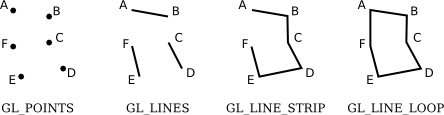The points A, B, C, D, E, and F were specified in that order. In this illustration, all the points lie in the same plane, but keep in mind that in general, points can be anywhere in 3D space.

The width for line primitives can be set by calling glLineWidth(width). The line width is always specified in pixels. It is not subject to scaling by transformations.

Let's look at an example. OpenGL does not have a circle primitive, but we can approximate a circle by drawing a polygon with a large number of sides. To draw an outline of the polygon, we can use a GL_LINE_LOOP primitive:

```glBegin( GL_LINE_LOOP );
for (i = 0; i < 64; i++) {
angle = 6.2832 * i / 64;  // 6.2832 represents 2*PI
x = 0.5 * cos(angle);
y = 0.5 * sin(angle);
glVertex2f( x, y );
}
glEnd();```

This draws an approximation for the circumference of a circle of radius 0.5 with center at (0,0). Remember that to learn how to use examples like this one in a complete, running program, you will have to read Section 3.6. Also, you might have to make some changes to the code, depending on which OpenGL implementation you are using.

The next set of primitives is for drawing triangles. There are three of them: GL_TRIANGLES, GL_TRIANGLE_STRIP, and GL_TRIANGLE_FAN.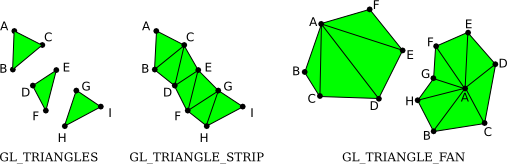The three triangles on the left make up one GL_TRIANGLES primitive, with nine vertices. With that primitive, every set of three vertices makes a separate triangle. For a GL_TRIANGLE_STRIP primitive, the first three vertices produce a triangle. After that, every new vertex adds another triangle to the strip, connecting the new vertex to the two previous vertices. Two GL_TRIANGLE_FAN primitives are shown on the right. Again for a GL_TRIANGLE_FAN, the first three vertices make a triangle, and every vertex after that adds anther triangle, but in this case, the new triangle is made by connecting the new vertex to the previous vertex and to the very first vertex that was specified (vertex "A" in the picture). Note that Gl_TRIANGLE_FAN can be used for drawing filled-in polygons. In this picture, by the way, the dots and lines are not part of the primitive; OpenGL would only draw the filled-in, green interiors of the figures.

The three remaining primitives, which have been removed from modern OpenGL, are GL_QUADS, GL_QUAD_STRIP, and GL_POLYGON. The name "quad" is short for quadrilateral, that is, a four-sided polygon. A quad is determined by four vertices. In order for a quad to be rendered correctly in OpenGL, all vertices of the quad must lie in the same plane. The same is true for polygon primitives. Similarly, to be rendered correctly, quads and polygons must be convex (see Subsection 2.2.3). Since OpenGL doesn't check whether these conditions are satisfied, the use of quads and polygons is error-prone. Since the same shapes can easily be produced with the triangle primitives, they are not really necessary, but here for the record are some examples: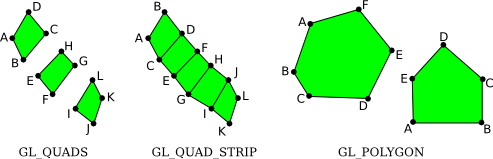The vertices for these primitives are specified in the order A, B, C, .... Note how the order differs for the two quad primitives: For GL_QUADS, the vertices for each individual quad should be specified in counterclockwise order around the quad; for GL_QUAD_STRIP, the vertices should alternate from one side of the strip to the other.

### 3.1.2  OpenGL Color

OpenGL has a large collection of functions that can be used to specify colors for the geometry that we draw. These functions have names of the form glColor*, where the "*" stands for a suffix that gives the number and type of the parameters. I should warn you now that for realistic 3D graphics, OpenGL has a more complicated notion of color that uses a different set of functions. You will learn about that in the next chapter, but for now we will stick to glColor*.

For example, the function glColor3f has three parameters of type float. The parameters give the red, green, and blue components of the color as numbers in the range 0.0 to 1.0. (In fact, values outside this range are allowed, even negative values. When color values are used in computations, out-of-range values will be used as given. When a color actually appears on the screen, its component values are clamped to the range 0 to 1. That is, values less than zero are changed to zero, and values greater than one are changed to one.)

You can add a fourth component to the color by using glColor4f(). The fourth component, known as alpha, is not used in the default drawing mode, but it is possible to configure OpenGL to use it as the degree of transparency of the color, similarly to the use of the alpha component in the 2D graphics APIs that we have looked at. You need two commands to turn on transparency:

```glEnable(GL_BLEND);
glBlendFunc(GL_SRC_ALPHA, GL_ONE_MINUS_SRC_ALPHA);```

The first command enables use of the alpha component. It can be disabled by calling glDisable(GL_BLEND). When the GL_BLEND option is disabled, alpha is simply ignored. The second command tells how the alpha component of a color will be used. The parameters shown here are the most common; they implement transparency in the usual way. I should note that while transparency works fine in 2D, it is much more difficult to use transparency correctly in 3D.

If you would like to use integer color values in the range 0 to 255, you can use glColor3ub() or glColor4ub to set the color. In these function names, "ub" stands for "unsigned byte." Unsigned byte is an eight-bit data type with values in the range 0 to 255. Here are some examples of commands for setting drawing colors in OpenGL:

```glColor3f(0,0,0);         // Draw in black.

glColor3f(1,1,1);         // Draw in white.

glColor3f(1,0,0);         // Draw in full-intensity red.

glColor3ub(1,0,0);        // Draw in a color just a tiny bit different from
// black.  (The suffix, "ub" or "f", is important!)

glColor3ub(255,0,0);      // Draw in full-intensity red.

glColor4f(1, 0, 0, 0.5);  // Draw in transparent red, but only if OpenGL
// has been configured to do transparency.  By
// default this is the same as drawing in plain red.```

Using any of these functions sets the value of a "current color," which is part of the OpenGL state. When you generate a vertex with one of the glVertex* functions, the current color is saved along with the vertex coordinates, as an attribute of the vertex. We will see that vertices can have other kinds of attribute as well as color. One interesting point about OpenGL is that colors are associated with individual vertices, not with complete shapes. By changing the current color between calls to glBegin() and glEnd(), you can get a shape in which different vertices have different color attributes. When you do this, OpenGL will compute the colors of pixels inside the shape by interpolating the colors of the vertices. (Again, since OpenGL is extremely configurable, I have to note that interpolation of colors is just the default behavior.) For example, here is a triangle in which the three vertices are assigned the colors red, green, and blue: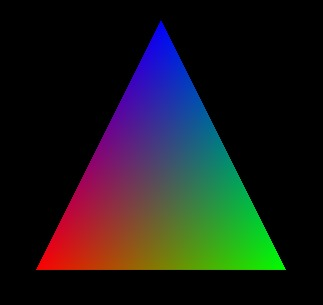This image is often used as a kind of "Hello World" example for OpenGL. The triangle can be drawn with the commands

```glBegin(GL_TRIANGLES);
glColor3f( 1, 0, 0 ); // red
glVertex2f( -0.8, -0.8 );
glColor3f( 0, 1, 0 ); // green
glVertex2f( 0.8, -0.8 );
glColor3f( 0, 0, 1 ); // blue
glVertex2f( 0, 0.9 );
glEnd();```

Note that when drawing a primitive, you do not need to explicitly set a color for each vertex, as was done here. If you want a shape that is all one color, you just have to set the current color once, before drawing the shape (or just after the call to glBegin(). For example, we can draw a solid yellow triangle with

```glColor3ub(255,255,0);  // yellow
glBegin(GL_TRIANGLES);
glVertex2f( -0.5, -0.5 );
glVertex2f( 0.5, -0.5 );
glVertex2f( 0, 0.5 );
glEnd();```

Also remember that the color for a vertex is specified before the call to glVertex* that generates the vertex.

Here is an interactive demo that draws the basic OpenGL triangle, with different colored vertices. You can control the colors of the vertices to see how the interpolated colors in the interior of the triangle are affected. This is our first OpenGL example. The demo actually uses WebGL, so you can use it as a test to check whether your web browser supports WebGL.

The sample program jogl/FirstTriangle.java draws the basic OpenGL triangle using Java. The program glut/first-triangle.c does the same using the C programming language. And glsim/first-triangle.html is a version that uses my JavaScript simulator, which implements just the parts of OpenGL 1.1 that are covered in this book. Any of those programs could be used to experiment with 2D drawing in OpenGL. And you can use them to test your OpenGL programming environment.

A common operation is to clear the drawing area by filling it with some background color. It is be possible to do that by drawing a big colored rectangle, but OpenGL has a potentially more efficient way to do it. The function

`glClearColor(r,g,b,a);`

sets up a color to be used for clearing the drawing area. (This only sets the color; the color isn't used until you actually give the command to clear the drawing area.) The parameters are floating point values in the range 0 to 1. There are no variants of this function; you must provide all four color components, and they must be in the range 0 to 1. The default clear color is all zeros, that is, black with an alpha component also equal to zero. The command to do the actual clearing is:

`glClear( GL_COLOR_BUFFER_BIT );`

The correct term for what I have been calling the drawing area is the color buffer, where "buffer" is a general term referring to a region in memory. OpenGL uses several buffers in addition to the color buffer. We will encounter the "depth buffer" in just a moment. The glClear command can be used to clear several different buffers at the same time, which can be more efficient than clearing them separately since the clearing can be done in parallel. The parameter to glClear tells it which buffer or buffers to clear. To clear several buffers at once, combine the constants that represent them with an arithmetic OR operation. For example,

`glClear( GL_COLOR_BUFFER_BIT | GL_DEPTH_BUFFER_BIT );`

This is the form of glClear that is generally used in 3D graphics, where the depth buffer plays an essential role. For 2D graphics, the depth buffer is generally not used, and the appropriate parameter for glClear is just GL_COLOR_BUFFER_BIT.

### 3.1.3  glColor and glVertex with Arrays

We have see that there are versions of glColor* and glVertex* that take different numbers and types of parameters. There are also versions that let you place all the data for the command in a single array parameter. The names for such versions end with "v". For example: glColor3fv, glVertex2iv, glColor4ubv, and glVertex3dv. The "v" actually stands for "vector," meaning essentially a one-dimensional array of numbers. For example, in the function call glVertex3fv(coords), coords would be an array containing at least three floating point numbers.

The existence of array parameters in OpenGL forces some differences between OpenGL implementations in different programming languages. Arrays in Java are different from arrays in C, and arrays in JavaScript are different from both. Let's look at the situation in C first, since that's the language of the original OpenGL API.

In C, arrays variables are a sort of variation on pointer variables, and arrays and pointers can be used interchangeably in many circumstances. In fact, in the C API, array parameters are actually specified as pointers. For example, the parameter for glVertex3fv is of type "pointer to float." The actual parameter in a call to glVertex3fv can be an array variable, but it can also be any pointer that points to the beginning of a sequence of three floats. As an example, suppose that we want to draw a square. We need two coordinates for each vertex of the square. In C, we can put all 8 coordinates into one array and use glVertex2fv to pull out the coordinates that we need:

```float coords[] = { -0.5, -0.5,  0.5, -0.5,  0.5, 0.5,  -0.5, 0.5 };

glBegin(GL_TRIANGLE_FAN);
glVertex2fv(coords);      // Uses coords and coords.
glVertex2fv(coords + 2);  // Uses coords and coords.
glVertex2fv(coords + 4);  // Uses coords and coords.
glVertex2fv(coords + 6);  // Uses coords and coords.
glEnd();```

This example uses "pointer arithmetic," in which coords + N represents a pointer to the N-th element of the array. An alternative notation would be &coords[N], where "&" is the address operator, and &coords[N] means "a pointer to coords[N]". This will all seem very alien to people who are only familiar with Java or JavaScript. In my examples, I will avoid using pointer arithmetic, but I will occasionally use address operators.

As for Java, the people who designed JOGL wanted to preserve the ability to pull data out of the middle of an array. However, it's not possible to work with pointers in Java. The solution was to replace a pointer parameter in the C API with a pair of parameters in the JOGL API—one parameter to specify the array that contains the data and one to specify the starting index of the data in the array. For example, here is how the square-drawing code translates into Java:

```float[] coords = { -0.5F, -0.5F,  0.5F, -0.5F,  0.5F, 0.5F,  -0.5F, 0.5F };

gl2.glBegin(GL2.GL_TRIANGLES);
gl2.glVertex2fv(coords, 0);  // Uses coords and coords.
gl2.glVertex2fv(coords, 2);  // Uses coords and coords.
gl2.glVertex2fv(coords, 4);  // Uses coords and coords.
gl2.glVertex2fv(coords, 6);  // Uses coords and coords.
gl2.glEnd();```

There is really not much difference in the parameters, although the zero in the first glVertex2fv is a little annoying. The main difference is the prefixes "gl2" and "GL2", which are required by the object-oriented nature of the JOGL API. I won't say more about JOGL here, but if you need to translate my examples into JOGL, you should keep in mind the extra parameter that is required when working with arrays.

For the record, here are the glVertex* and glColor* functions that I will use in this book. This is not the complete set that is available in OpenGL:

```glVertex2f( x, y );             glVertex2fv( xyArray );
glVertex2d( x, y );             glVertex2dv( xyArray );
glVertex2i( x, y );             glVertex2iv( xyArray );
glVertex3f( x, y, z );          glVertex3fv( xyzArray );
glVertex3d( x, y, z );          glVertex3dv( xyzArray );
glVertex3i( x, y, z );          glVertex3iv( xyzArray );

glColor3f( r, g, b );           glColor3f( rgbArray );
glColor3d( r, g, b );           glColor3d( rgbArray );
glColor3ub( r, g, b );          glColor3ub( rgbArray );
glColor4f( r, g, b, a);         glColor4f( rgbaArray );
glColor4d( r, g, b, a);         glColor4d( rgbaArray );
glColor4ub( r, g, b, a);        glColor4ub( rgbaArray );```

For glColor*, keep in mind that the "ub" variations require integers in the range 0 to 255, while the "f" and "d" variations require floating-point numbers in the range 0.0 to 1.0.

### 3.1.4  The Depth Test

An obvious point about viewing in 3D is that one object can be behind another object. When this happens, the back object is hidden from the viewer by the front object. When we create an image of a 3D world, we have to make sure that objects that are supposed to be hidden behind other objects are in fact not visible in the image. This is the hidden surface problem.

The solution might seem simple enough: Just draw the objects in order from back to front. If one object is behind another, the back object will be covered up later when the front object is drawn. This is called the painter's algorithm. It's essentially what you are used to doing in 2D. Unfortunately, it's not so easy to implement. First of all, you can have objects that intersect, so that part of each object is hidden by the other. Whatever order you draw the objects in, there will be some points where the wrong object is visible. To fix this, you would have to cut the objects into pieces, along the intersection, and treat the pieces as separate objects. In fact, there can be problems even if there are no intersecting objects: It's possible to have three non-intersecting objects where the first object hides part of the second, the second hides part of the third, and the third hides part of the first. The painter's algorithm will fail regardless of the order in which the three objects are drawn. The solution again is to cut the objects into pieces, but now it's not so obvious where to cut.

Even though these problems can be solved, there is another issue. The correct drawing order can change when the point of view is changed or when a geometric transformation is applied, which means that the correct drawing order has to be recomputed every time that happens. In an animation, that would mean for every frame.

So, OpenGL does not use the painter's algorithm. Instead, it uses a technique called the depth test. The depth test solves the hidden surface problem no matter what order the objects are drawn in, so you can draw them in any order you want! The term "depth" here has to do with the distance from the viewer to the object. Objects at greater depth are farther from the viewer. An object with smaller depth will hide an object with greater depth. To implement the depth test algorithm, OpenGL stores a depth value for each pixel in the image. The extra memory that is used to store these depth values makes up the depth buffer that I mentioned earlier. During the drawing process, the depth buffer is used to keep track of what is currently visible at each pixel. When a second object is drawn at that pixel, the information in the depth buffer can be used to decide whether the new object is in front of or behind the object that is currently visible there. If the new object is in front, then the color of the pixel is changed to show the new object, and the depth buffer is also updated. If the new object is behind the current object, then the data for the new object is discarded and the color and depth buffers are left unchanged.

By default, the depth test is not turned on, which can lead to very bad results when drawing in 3D. You can enable the depth test by calling

`glEnable( GL_DEPTH_TEST );`

It can be turned off by calling glDisable(GL_DEPTH_TEST). If you forget to enable the depth test when drawing in 3D, the image that you get will likely be confusing and will make no sense physically. You can also get quite a mess if you forget to clear the depth buffer, using the glClear command shown earlier in this section, at the same time that you clear the color buffer.

Here is a demo that lets you experiment with the depth test. It also lets you see what happens when part of your geometry extends outside the visible range of z-values.

Here are are a few details about the implementation of the depth test: For each pixel, the depth buffer stores a representation of the distance from the viewer to the point that is currently visible at that pixel. This value is essentially the z-coordinate of the point, after any transformations have been applied. (In fact, the depth buffer is often called the "z-buffer".) The range of possible z-coordinates is scaled to the range 0 to 1. The fact that there is only a limited range of depth buffer values means that OpenGL can only display objects in a limited range of distances from the viewer. A depth value of 0 corresponds to the minimal distance; a depth value of 1 corresponds to the maximal distance. When you clear the depth buffer, every depth value is set to 1, which can be thought of as representing the background of the image.

You get to choose the range of z-values that is visible in the image, by the transformations that you apply. The default range, in the absence of any transformations, is -1 to 1. Points with z-values outside the range are not visible in the image. It is a common problem to use too small a range of z-values, so that objects are missing from the scene, or have their fronts or backs cut off, because they lie outside of the visible range. You might be tempted to use a huge range, to make sure that the objects that you want to include in the image are included within the range. However, that's not a good idea: The depth buffer has a limited number of bits per pixel and therefore a limited amount of accuracy. The larger the range of values that it must represent, the harder it is to distinguish between objects that are almost at the same depth. (Think about what would happen if all objects in your scene have depth values between 0.499999 and 0.500001—the depth buffer might see them all as being at exactly the same depth!)

There is another issue with the depth buffer algorithm. It can give some strange results when two objects have exactly the same depth value. Logically, it's not even clear which object should be visible, but the real problem with the depth test is that it might show one object at some points and the second object at some other points. This is possible because numerical calculations are not perfectly accurate. Here an actual example: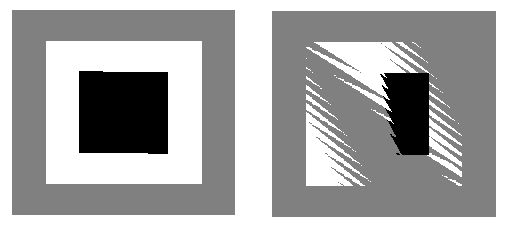In the two pictures shown here, a gray square was drawn, followed by a white square, followed by a black square. The squares all lie in the same plane. A very small rotation was applied, to force the computer do some calculations before drawing the objects. The picture on the left was drawn with the depth test disabled, so that, for example, when a pixel of the white square was drawn, the computer didn't try to figure out whether it lies in front of or behind the gray square; it simply colored the pixel white. On the right, the depth test was enabled, and you can see the strange result.

Finally, by the way, note that the discussion here assumes that there are no transparent objects. Unfortunately, the depth test does not handle transparency correctly, since transparency means that two or more objects can contribute to the color of the pixel, but the depth test assumes that the pixel color is the color of the object nearest to the viewer at that point. To handle 3D transparency correctly in OpenGL, you pretty much have to resort to implementing the painter's algorithm by hand, at least for the transparent objects in the scene.

[ Next Section | Chapter Index | Main Index ]